Click to Chat

1800-1023-196

+91-120-4616500

CART 0

• 0

MY CART (5)

Use Coupon: CART20 and get 20% off on all online Study Material

ITEM
DETAILS
MRP
DISCOUNT
FINAL PRICE
Total Price: Rs.

There are no items in this cart.
Continue Shopping• Complete Physics Course - Class 11
• OFFERED PRICE: Rs. 2,968
• View Details

Satellites and Planetary Motion

Table of Content

Earth and Its Satellite

Simulation for Kepler Laws of Motion

Kepler's Laws- Elliptical Motion of Planets and Satellites

Kepler's Second Law

Related Resources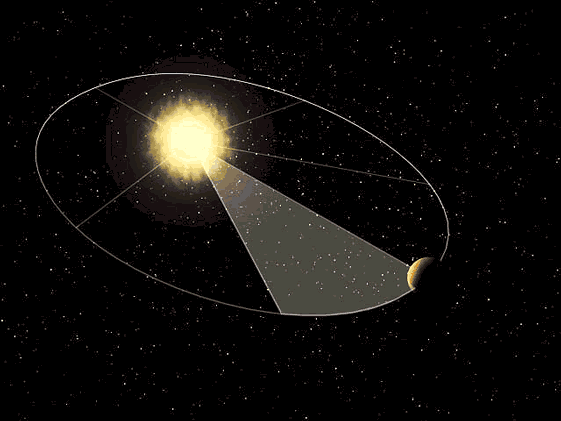It was Copernicus who, first of all, introduced the idea that the central body of our planetary system was Sun rather than Earth. Kepler later on confirmed by putting it on a solid mathematical back ground. Kepler announced two of his laws in 1609 and the third one 10 years later. The laws can be stated as below:

(a) Kepler’s first law (law of elliptical orbit):- A planet moves round the sun in an elliptical orbit with sun situated at one of its foci.

(b) Kepler’s second law (law of areal velocities):- A planet moves round the sun in such a way that its areal velocity is constant.

(c) Kepler’s third law (law of time period):- A planet moves round the sun in such a way that the square of its period is proportional to the cube of semi major axis of its elliptical orbit.

T2 ∝ r 3

Here R is the radius of orbit.

T2 = (4π2/GM) r 3

Earth and Its Satellite

Consider a satellite of mass m revolving in a circular orbit around the Earth, which is located at the centre of its orbit. If the satellite is at a height h above the Earth's surface, the radius of its orbit r = Re + h, where Re is the radius of the Earth. The gravitational force between Me & m provides the centripetal force necessary for circular motion, i.e.,

GMem/(Re+h)2    =  mv2/(Re+h)

Or v2 = GMe/(Re+h)     or     v = √GMe/(Re+h)

Hence orbital velocity depends on the height of the satellite above Earth's surface. Time period T of the satellite is the time taken to complete one revolution.

Therefore, T = 2Πr/v = 2Π(Re + h)√(Re+h)/GMe

or T2 = 4Π2(Re+h)3/GMe where r = Re + h

If time period of a satellite is 24 hrs. Then,

r = [GMeT2/4Π2]1/3 = 42400 km and h = 36000 km.

This gives the height of a satellite above the Earth's surface whose time period is same as that of Earth's. Such a satellite appears to be stationary when observed from the Earth's surface and is hence known as Geostationary satellite.

For a satellite very close to the surface of Earth i.e. h << Re then

r ≈ Re

vorbital = √GMe/Re = √gRe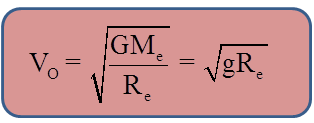Simulation for Kepler Laws of Motion

Satellite in circular orbit:-

For different velocities, the trajectory of the satellite would be different. Let us consider these cases.

If v is the velocity given to a satellite and vO represents the velocity of a circular orbit and ve the escape velocity.

i.e. v0 = √GMe/(Re+h)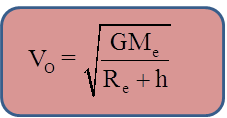ve =  √2GMe/(Re+h)

Where, h is the distance of the satellite from the surface of the Earth.Note:

When, v < v0, the satellite follows an elliptical path with center of the Earth as the further focus. In this case, if satellite is projected from near surface of the Earth, it will hit the Earth's surface without completing the orbit.

If v = v0, obviously the satellite follows a circular orbit with center of Earth as the center of the orbit.

If v0 < v < ve, then the satellite follows an elliptical orbit with center of the Earth as the nearer focus.

If v = ve, the satellite escapes the gravitational field of the Earth along a parabolic trajectory.

If v > ve, the satellite escapes the gravitational field of Earth along a hyperbolic trajectory

Question:-

What should be the energy required to shift a satellite orbiting around the Earth to infinity?

At infinity the Potential Energy of the satellite would be zero and if we want to supply minimum Energy then its kinetic energy would also be zero. Let us first find the total Energy of the satellite.

Total Energy = Kinetic Energy + Potential Energy

= 1/2 mv2 + (-GMem/r)

= 1/2 m (GMe/r) - GMem/r

= -GMem/2r

Now, Binding Energy would be equal to - (Total Energy) as it is the energy needed to shift the satellite from its orbit to infinity.

So, the energy required = GMem/2r.

Here,  r = Re + h.

Question:-

If we see a satellite from Earth, how long will it take for one revolution?

Let us consider a satellite in circular orbit with a time period Ts. The Earth also rotates with the time period Te = 24 hrs. If an observer on Earth sees this satellite, the angular velocity of the satellite will be . Hence, the time period of revolution will seem different from Ts and will be observed as TSE.

Two cases arise for calculation of TSE:-

(i) If the satellite and Earth are revolving and rotating respectively in the same direction.

Hence, 2Π/TSE = |2Π/Ts - 2Π/Te|

=> TSE = TsTe/|Te - Ts|

(ii) If the satellite and Earth are revolving and rotating respectively in the opposite direction.

Hence,2Π/TSE = 2Π/Ts  + 2Π/Te

or TSE =  TsTe/|Ts - Te|.

Kepler's Laws- Elliptical Motion of Planets and Satellites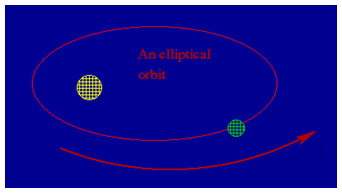One of the greatest ideas proposed in human history is the fact that the earth is a planet, among the other planets, that orbits the sun. The precise determination of these planetary orbits was carried out by Jhannes Kepler, using the data compiled by his teacher, the astronomer Tycho Brahe. Johannes Kelper discovered three empirical laws by using the data on planetary motion.

(a) Each planet moves in an elliptical orbit, with the sun at one foci of the ellipse.

(b) A line from the sun to a given planet sweeps out equal areas in equal intervals of time.

(c) The square of the periods of the planets are proportional to cube of their mean distance (or semi-major axis) from the sun.

These laws go by the name 'Kepler's laws of planetary motion'. It was in order to explain the origin of these laws, among other phenomena, that Newton proposed the theory of gravitation.

In our discussion, we are not going to derive the complete laws of planetary motion from Newton's law of gravitation. Since most of the planets actually revolve in near circular orbits, we're going to assume that the planets revolve in circular orbits.

Refer this video for better understanding about kepler’s laws of motion:-

Consider a planet of mass m rotating around the sun (mass M >> m) in a circular orbit of radius r with velocity v. Then, by applying Newton's law of gravitation and the second law of motion, we can write

Gravitational force = mass × centripetal acceleration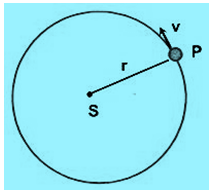i.e. GMm/r2  = m(v2/r)                                             ... (1)

or, v2 = GMm/r                                                       ... (2)

As the moment of the gravitational force about S is zero, the angular momentum of the planet about the sun remains constant. This is the meaning of Kepler's 2nd law of motion, as will be shown later.

The time period of rotation, T, of the planet around the sun is given by,

T = 2Πr/v = -2Πr/√GM/r = 2Π/√GM r3/2                         ... (3)

Squaring both sides,

T2 = (4Π2/GM)r3                                                        ... (4)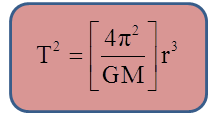which is Kepler's 3rd law of motion.Note:

The constant of proportionality in the above equation depends only on the mass of the sun (M) but not on the mass of the planet.

Kepler's Laws are also valid for the motion satellites around the earth.

Kepler's Second Law

Consider a planet P that moves in an elliptical orbit around the sun, and let P and P' be the positions of the planet at time t and t + Δt (where Δt is a very small time interval). If the angular displacement of the planet is Δθ, then the are swept out by the line joining the planet and sun (SP) in time Δt is:ΔA = area of the section SPP'

= 1/2 r2.Δθ;    where r = the length SP.

The area velocity, vA = ΔA/Δt = 1/2r2Δθ/Δt = 1/2r2ω = constant    .... (5)

In other words,

m × (2vA) = constant as well (m = mass of the planet)

Areal velocity = dA/dt = L/2m                                            ... (6)

This is the expression for the angular momentum of the planet,

L = Iω = mr2ω

= mr2 (dθ/dt) perpendicular to the plane of its orbit.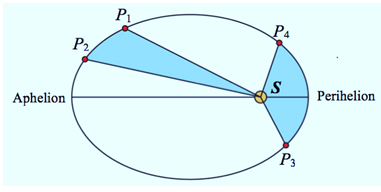The gravitational force,= -GMm/r2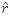is centripetal, and the torque on the planet is zero,

So,

Hence, the angular momentum of the planet does not vary, i.e. the areal velocity of the planet remains constant. At its aphelion (farthest point from the sun, r is large), the planet moves slowly and at its perihelion (nearest point from the sun, r is small) the planet moves fastest.The  gravitational force between two particles are equal in magnitude but oppositely directed.

The gravitational force between two particles does not depend on the presence of the other bodies.

The gravitational force between two particles does not depend on the nature of the medium between the particles.

The force of gravitation is expressed in terms of the force between the masses of particles.

The value of gravitational force in the case of small bodies is very small but is appreciable in the case of massive bodies.

Problem 1:-

Calculate the mass of the Sun from the following data; distance between the Sun and the Earth = 1.49 × 1011 m, G = 6.67 × 10-11 SI units and one year = 365 days.

Solution:-

Force of attraction between the sun and the earth = GmsmE/dSE2

Considering the orbit of the earth as nearly circular, the centripetal force acting on the earth is mE dSEω2.

=> mE dSE ω2 = GmsmE/dSE2

mS = dSE2.ω2/G = 4Π2dSE2/GT2

=(4×(3.14)2×(1.49×1011)2)/(6.67×10-11×(365×24×60×60)2)

=1.32 × 1019 kg.

Problem 2:-

A Saturn year is 29.5 times the earth year. How far is Saturn from the sun (M) if the earth is 1.5 × 108 km away from the sun?

Solution:-

It is given that

TS = 29.5 Te;              Re = 1.5 × 1011 m

Now, according to kepler's third law

TS2/Te2 = Rs3/Re3

RS=Re(TS/Te)2/3=1.5×1011 ((29.5Te)/Te)2⁄3=1.43×1012 m =1.43×109 km

Problem 3:-

A planet of mass m moves along an ellipse around the sun so that its maximum and minimum distances form the sun are equal to R and r, respectively. Find the angular momentum of this planet relative to the center of the sun.

Solution:-

According to Kepler's second law, the angular momentum of the planet is constant.

So, mv1R = mv2r or v1R = v2r

If the mass of the Sun is M, conserving total mechanical energy of the system at two given positions we have,-GMm/R + 1/2 mv12 = -(G M m)/r + 1/2 mv22

So, GM[1/R - 1/r] = v12/2 + v22/2 or GM[(r-R)/Rr] = v12/2 + v22R2/2r2

Thus, v12= (2GM(R-r) r2)/Rr(R2-r2 ) = 2GMr/R(R+r)

Now, angular momentum = mv1R = m√2GMR/(R+r)Question 1:-

Gravitational forces are:

(a) always attractive

(b) always repulsive

(c) sometimes attractive and sometimes repulsive

(d) none

Question 2:-

The atmosphere is held to the earth by:

(a) winds      (b) gravity     (c) clouds      (d) the rotation of the earth

Question 3:-

Weight of the body is maximum:

(a) on the poles

(b) on the equator

(c) at a place in between pole and equator

(d) none

Question 4:-

A body mass m is taken to the bottom of a deep mine. Then :

(a) its mass increases

(b) its mass decreases

(c) its weight increases

(d) its weight decreases

Question 5:-

If Ve and VP denote the escape velocities from the earth and from another planet having twice the radius and the same mean density as the earth. Then:

(a) Ve = VP     (b) Ve = VP/2     (c) Ve = 2VP    (d) Ve = VP/4Q.1
Q.2
Q.3
Q.4
Q.5

a

b

a

d

b

Related Resources

You might like to refer Gravitational Potential Energy.

For getting an idea of the type of questions asked, refer the  Previous Year Question Papers.### Course Features

• 101 Video Lectures
• Revision Notes
• Previous Year Papers
• Mind Map
• Study Planner
• NCERT Solutions
• Discussion Forum
• Test paper with Video Solution